# How to increase font size in Base R Plot ?

The plot() method in the R programming language is used to plot a series of points in the graph and visualize them using curves and scatter that they follow.

Syntax:

plot(x, y, main=”title”, sub=”subtitle”)

The cex attribute is the integer which is an indicator of the scale factor, which describes the amount by which the elements of the plot can be scaled. The default value is 1, 0.5 reduces it by 50%, etc. The cex parameter may have whole numbers or decimal values.

The main title can be added in the plot to convey more features using the main option in the plot method, whereas, the subtitle can be added using the sub-attribute. The size of the main title heading can be modified using the cex.main option and subtitle using the cex.sub option respectively.

The following graph has been used for reference to create the different components scaled to font size = 1 by default. It displays the graph for the equation y = x2, where the x values have been created using the seq() function in R. This helps to understand the graph better and the difference is also apparent.

Example:

## R

 `# declaring a sequence of integers ` `xpos <- ``seq``(0.1 , length.out = 50,by = 0.1) ` ` `  `# computing its square ` `ypos <- xpos**2 ` ` `  `main_title <- ``"Squares of numbers"` ` `  `# plotting the data ` `plot``(xpos, ypos, main = main_title)`

Output: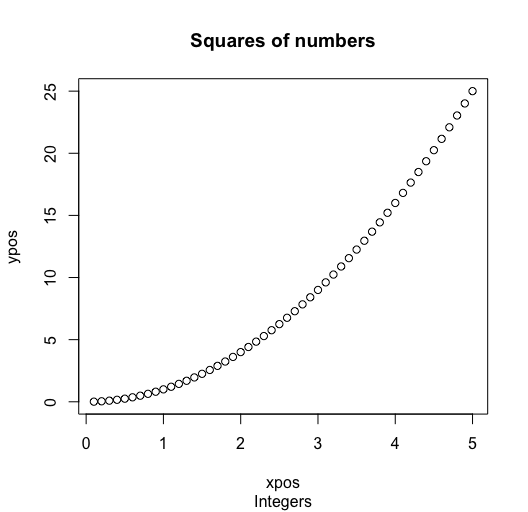### Increasing the font size of labels

The cex.lab attribute can be used to modify the font size of the labels of the both (x and y) axes. The following code snippet illustrates the procedure of making labels’ font size double.

Example:

## R

 `# declaring a sequence of integers ` `xpos <- ``seq``(0.1 , length.out = 50,by = 0.1) ` ` `  `# computing its square ` `ypos <- x**2 ` ` `  `main_title <- ``"Squares of numbers"` ` `  `# plotting the data ` `plot``(xpos, ypos, main = main_title, cex.lab = 2)`

Output: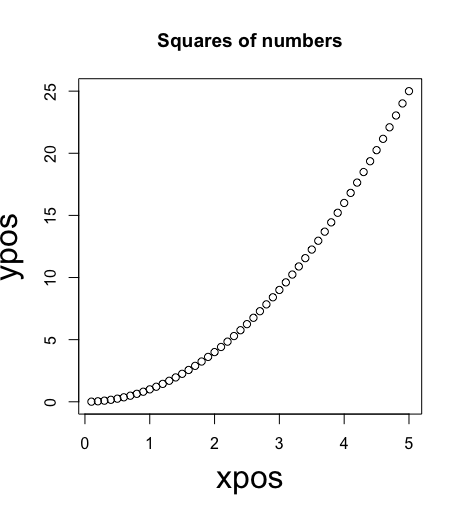### Increasing the font size of axes

The cex.axes attribute can be used to modify the font size of the axis tick labels. Just pass the value you want the font to be increased with.

Example:

## R

 `# declaring a sequence of integers ` `xpos <- ``seq``(0.1 , length.out = 50,by = 0.1) ` ` `  `# computing its square ` `ypos <- xpos**2 ` ` `  `main_title <- ``"Squares of numbers"` ` `  `# plotting the data ` `plot``(xpos, ypos, main = main_title, cex.axes = 2)`

Output: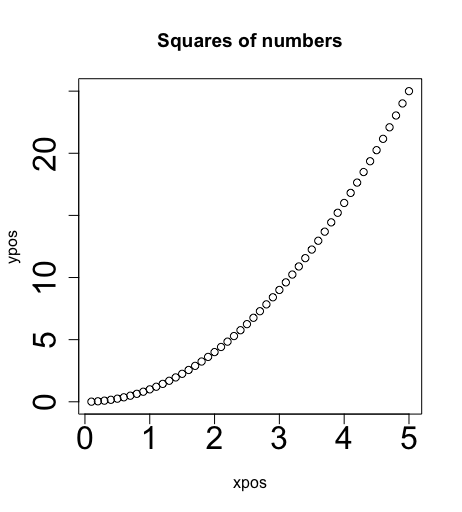### Increasing the font size of main title

The cex.main attribute can be used to modify the font size of the main title specified for the graph.

Example:

## R

 `# declaring a sequence of integers ` `xpos <- ``seq``(0.1 , length.out = 50,by = 0.1) ` ` `  `# computing its square ` `ypos <- xpos**2 ` ` `  `main_title <- ``"Squares of numbers"` ` `  `# plotting the data ` `plot``(xpos, ypos, main = main_title, cex.main = 2)`

Output: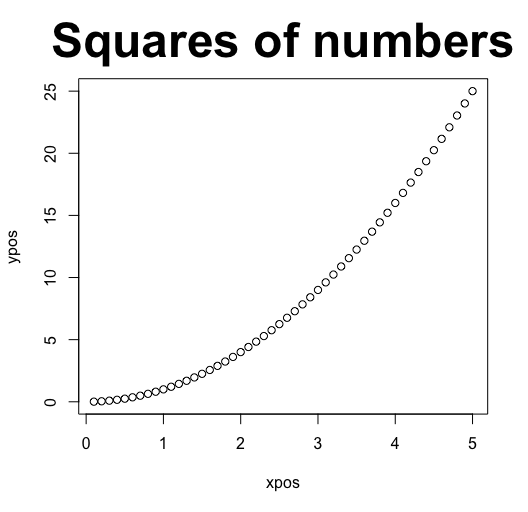### Increasing the size of sub heading

The cex.sub attribute can be used to modify the font size of the subtitle specified for the graph.

Example:

## R

 `# declaring a sequence of integers ` `xpos <- ``seq``(0.1 , length.out = 50,by = 0.1) ` ` `  `# computing its square ` `ypos <- xpos**2 ` ` `  `main_title <- ``"Squares of numbers"` `sub_title <- ``"Integers"` ` `  `# plotting the data ` `plot``(xpos, ypos, main = main_title , sub= sub_title , cex.sub = 2)`

Output: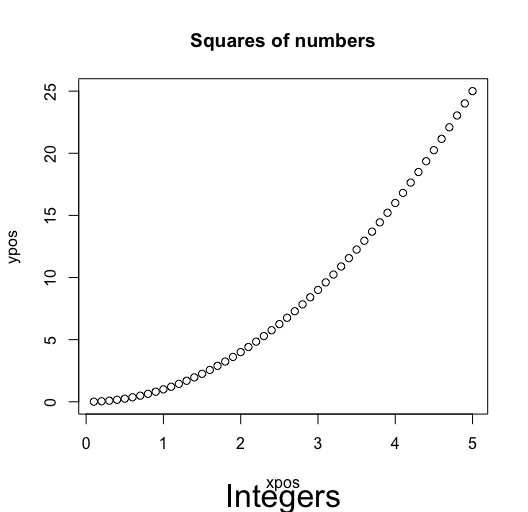### Increasing all the properties of the graph

To increase the size of properties, all the properties with their respective values are passed to the function.

Example:

## R

 `# declaring a sequence of integers ` `xpos <- ``seq``(0.1 , length.out = 50,by = 0.1) ` ` `  `# computing its square ` `ypos <- xpos**2 ` ` `  `main_title <- ``"Squares of numbers"` `sub_title <- ``"Integers"` ` `  `# plotting the data ` `plot``(xpos, ypos, main = main_title , sub= sub_title , cax.lab = 1.9,  ` `     ``cex.axes = 1.52, cex.main = 2.5, cex.sub = 2)`

Output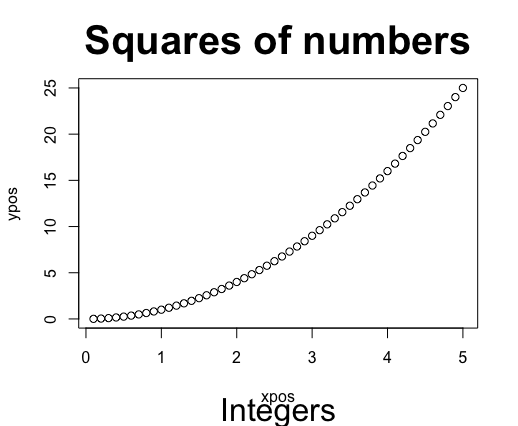Whether you're preparing for your first job interview or aiming to upskill in this ever-evolving tech landscape, GeeksforGeeks Courses are your key to success. We provide top-quality content at affordable prices, all geared towards accelerating your growth in a time-bound manner. Join the millions we've already empowered, and we're here to do the same for you. Don't miss out - check it out now!

Previous
Next